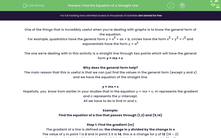# Find the Equation of a Straight Line

In this worksheet, students will practise finding the equation of a straight line when they are given two points.Key stage:  KS 4

Year:  GCSE

GCSE Subjects:   Maths

GCSE Boards:   AQA, Eduqas, OCR, Pearson Edexcel,

Curriculum topic:   Algebra, Graphs of Equations and Functions

Curriculum subtopic:   Graphs Graphs of Equations and Functions

Difficulty level:#### Worksheet Overview

One of the things that is incredibly useful when you’re dealing with graphs is to know the general form of the equation.

For example, quadratics have the general form y = x2 + ax + b, circles have the form x2 + y2 = r2 and exponentials have the form y = ax

The one we're dealing with in this activity is a straight line through two points which will have the general form y = mx + c

Why does the general form help?

The main reason that this is useful is that we can just find the values in the general form (except y and x) and we have the equation of the straight line.

y = mx + c

Hopefully, you  know from earlier in your studies that in the equation y = mx + c, m represents the gradient and c represents the y-intercept.

All we have to do is find m and c.

Example:

Find the equation of a line that passes through (1,2) and (5,14)

Step 1: Find the gradient (m)

The gradient of a line is defined as: the change in y divided by the change in x

The value of y in point 1 is 2 and in point 2 it is 14, this is a change for y of 12 (14 – 2)

The value of x in point 1 is 1 and in point 2 it is 5, this is a change for y of 4 (5 – 1)

Our gradient will therefore be 12 ÷ 4 = 3

We can now write the first part of the equation of the line: y = 3x + c

Step 2: Find the intercept (c)

So far, we have the equation y = 3x + c

To find the value of c, we have to know the values of y and x. Luckily, we have two points that we know will work (1,2) and (5,14).

It is usually easier to use the smaller numbers (1,2), but you can use (5,14) if you want - you’ll get the same answers.

y = 3x + c

(2) = 3 x (1) + c

2 = 3 + c

c = - 1

We can now put all of this together and get the equation of the straight line:

y = 3x - 1

Let's move on to some questions now.

### What is EdPlace?

We're your National Curriculum aligned online education content provider helping each child succeed in English, maths and science from year 1 to GCSE. With an EdPlace account you’ll be able to track and measure progress, helping each child achieve their best. We build confidence and attainment by personalising each child’s learning at a level that suits them.

Get started